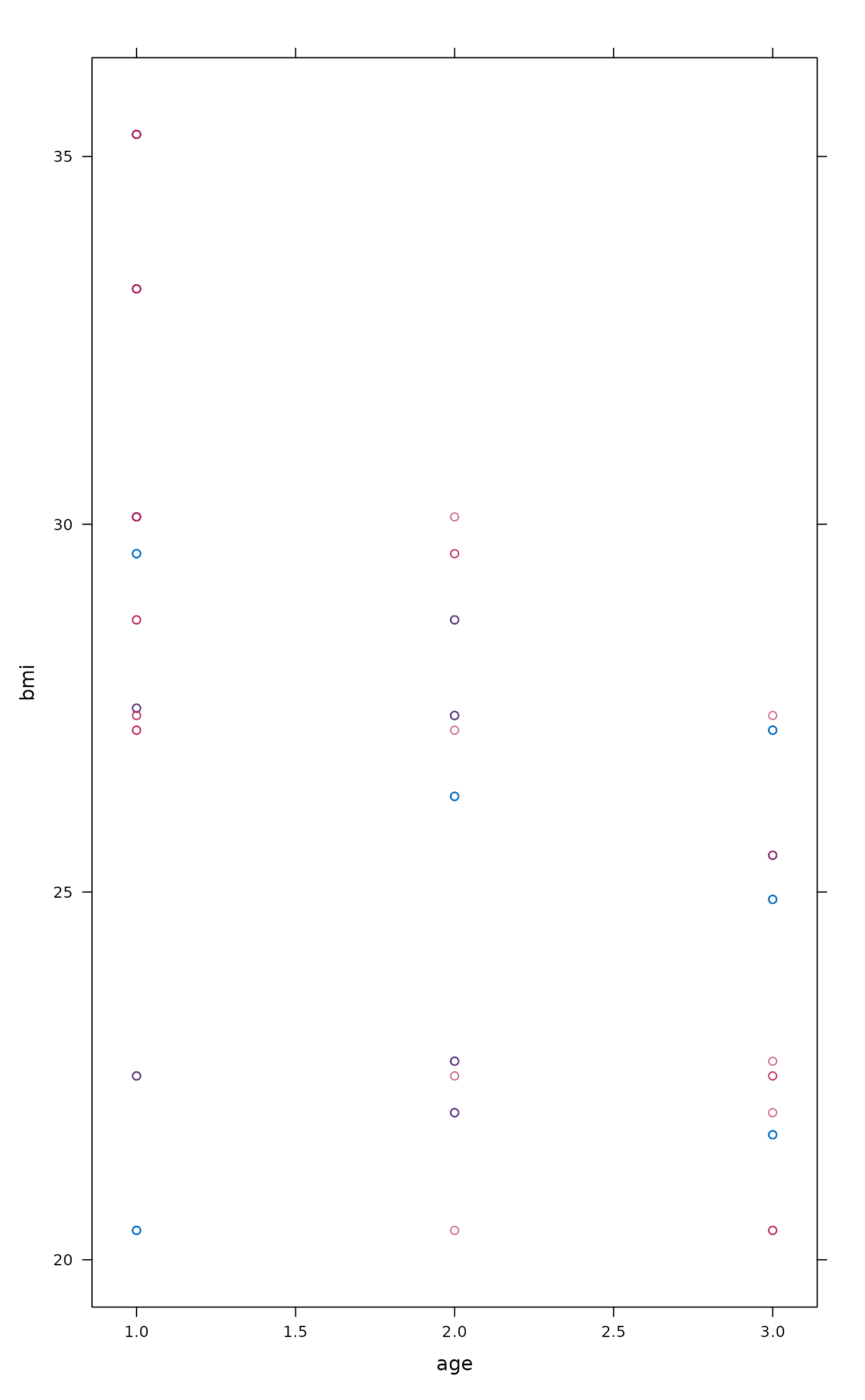Scatterplot of observed and imputed data

## Usage

xyplot(...)

## Arguments

...

Any arguments passed to the function.

## Value

The output of mice::xyplot and a message about the ggmice equivalent.

## Examples

imp <- mice::mice(mice::nhanes, maxit = 1, printFlag = FALSE)
xyplot(imp, bmi ~ age)
#> Hint: Did you know, an equivalent figure can be created with ggmice()?
#> For example, to plot 2 variables named 'my_x' and 'my_y' from a mids object
#> called 'my_mids', run:
#>   ggmice(my_mids, ggplot2::aes(x = my_x, y = my_y)) +
#>   ggplot2::geom_point()